### PROC SURVEYMEANS Statement

PROC SURVEYMEANS <options> statistic-keywords ;

The PROC SURVEYMEANS statement invokes the SURVEYMEANS procedure. In this statement, you identify the data set to be analyzed, specify the variance estimation method, and provide sample design information. The DATA= option names the input data set to be analyzed. The VARMETHOD= option specifies the variance estimation method, which is the Taylor series method by default. For Taylor series variance estimation, you can include a finite population correction factor in the analysis by providing either the sampling rate or population total with the RATE= or TOTAL= option. If your design is stratified, with different sampling rates or totals for different strata, then you can input these stratum rates or totals in a SAS data set that contains the stratification variables.

In the PROC SURVEYMEANS statement, you also can use statistic-keywords to specify statistics, such as population mean and population total, for the procedure to compute. You can also request data set summary information and sample design information.

Table 92.2 summarizes the options available in the PROC SURVEYMEANS statement.

Table 92.2: PROC SURVEYMEANS Statement Options

Option

Description

Sets the confidence level for confidence limits

Specifies the SAS data set to be analyzed

Treats missing values as a valid

Computes variance estimates by analyzing the nonmissing values as a domain

Requests nonsymmetric confidence limits for quantiles

Suppresses the display of analysis variables with zero frequency

Specifies the order in which to report the values of the categorical variables

Specifies percentiles that you want the procedure to compute

Specifies quantiles that you want the procedure to compute

Specifies the sampling rate

Produces the output data sets by using a stacking table structure

Specifies the total number of primary sampling units

Specifies the variance estimation method

You can specify the following options in the PROC SURVEYMEANS statement:

ALPHA=sets the confidence level for confidence limits. The value of the ALPHA= option must be between 0 and 1, and the default value is 0.05. A confidence level ofproduces% confidence limits. The default of ALPHA=0.05 produces 95% confidence limits.

DATA=SAS-data-set

specifies the SAS data set to be analyzed by PROC SURVEYMEANS. If you omit the DATA= option, the procedure uses the most recently created SAS data set.

MISSING

treats missing values as a valid (nonmissing) category for all categorical variables, which include CLASS, STRATA, CLUSTER, DOMAIN, and POSTSTRATA variables.

By default, if you do not specify the MISSING option, an observation is excluded from the analysis if it has a missing value. For more information, see the section Missing Values.

NOMCAR

requests that the procedure treat missing values in the variance computation as not missing completely at random (NOMCAR) for Taylor series variance estimation. When you specify the NOMCAR option, PROC SURVEYMEANS computes variance estimates by analyzing the nonmissing values as a domain (subpopulation), where the entire population includes both nonmissing and missing domains. See the section Missing Values for more details.

By default, PROC SURVEYMEANS completely excludes an observation from analysis if that observation has a missing value, unless you specify the MISSING option for categorical variables. Note that the NOMCAR option has no effect on a categorical variable when you specify the MISSING option, which treats missing values as a valid nonmissing level.

The NOMCAR option applies only to Taylor series variance estimation. The replication methods, which you request with the VARMETHOD=BRR and VARMETHOD=JACKKNIFE options, do not use the NOMCAR option.

The NOMCAR option is not available for geometric means or poststratification.

NONSYMCL

requests nonsymmetric confidence limits for quantiles when you request quantiles with PERCENTILE= or QUANTILE= option. See the section Confidence Limits for more details. This option applies only to the default VARMETHOD=TAYLOR option.

NOSPARSE

suppresses the display of analysis variables with zero frequency. By default, the procedure displays all continuous variables and all levels of categorical variables.

ORDER=DATA | FORMATTED | INTERNAL

specifies the order in which the values of the categorical variables are to be reported.

This option also determines the sort order for the levels of ClUSTER and DOMAIN variables and controls STRATA variable levels in the Stratum Information table.

The following shows how PROC SURVEYMEANS interprets values of the ORDER= option:

DATA

orders values according to their order in the input data set.

FORMATTED

orders values by their formatted values. This order is operating environment dependent. By default, the order is ascending.

INTERNAL

orders values by their unformatted values, which yields the same order that the SORT procedure does. This order is operating environment dependent.

By default, ORDER=INTERNAL. For ORDER=FORMATTED and ORDER=INTERNAL, the sort order is machine-dependent.

For more information about sort order, see the chapter on the SORT procedure in the Base SAS Procedures Guide and the discussion of BY-group processing in SAS Language Reference: Concepts.

PERCENTILE=(values)

specifies percentiles you want the procedure to compute. You can separate values with blanks or commas. Each value must be between 0 and 100. You can also use the statistic-keywords DECILES, MEDIAN, Q1, Q3, and QUARTILES to request common percentiles.

PROC SURVEYMEANS uses Woodruff’s method (Dorfman and Valliant, 1993; Särndal, Swensson, and Wretman, 1992; Francisco and Fuller, 1991) to estimate the variances of quantiles. See the section Quantiles for more details.

QUANTILE=(values)

specifies quantiles you want the procedure to compute. You can separate values with blanks or commas. Each value must be between 0 and 1. You can also use the statistic-keywords DECILES, MEDIAN, Q1, Q3, and QUARTILES to request common quantiles.

PROC SURVEYMEANS uses Woodruff’s method (Dorfman and Valliant, 1993; Särndal, Swensson, and Wretman, 1992; Francisco and Fuller, 1991) to estimate the variances of quantiles. See the section Quantiles for more details.

RATE=value | SAS-data-set
R=value | SAS-data-set

specifies the sampling rate as a nonnegative value, or specifies an input data set that contains the stratum sampling rates. The procedure uses this information to compute a finite population correction for Taylor series variance estimation. The procedure does not use the RATE= option for BRR or jackknife variance estimation, which you request with the VARMETHOD=BRR or VARMETHOD=JACKKNIFE option.

If your sample design has multiple stages, you should specify the first-stage sampling rate, which is the ratio of the number of PSUs selected to the total number of PSUs in the population.

For a nonstratified sample design, or for a stratified sample design with the same sampling rate in all strata, you should specify a nonnegative value for the RATE= option. If your design is stratified with different sampling rates in the strata, then you should name a SAS data set that contains the stratification variables and the sampling rates. See the section Specification of Population Totals and Sampling Rates for more details.

The value in the RATE= option or the values of _RATE_ in the secondary data set must be nonnegative numbers. You can specify value as a number between 0 and 1. Or you can specify value in percentage form as a number between 1 and 100, and PROC SURVEYMEANS converts that number to a proportion. The procedure treats the value 1 as 100% instead of 1%.

If you do not specify the TOTAL= or RATE= option, then the Taylor series variance estimation does not include a finite population correction. You cannot specify both the TOTAL= and RATE= options.

STACKING

requests that the procedure produce the output data sets by using a stacking table structure, which was the default before SAS 9. The new default is to produce a rectangular table structure in the output data sets.

A rectangular structure creates one observation for each analysis variable in the data set. A stacking structure creates only one observation in the output data set for all analysis variables.

The STACKING option affects the following tables:

• Domain

• Ratio

• Statistics

• StrataInfo

See the section Rectangular and Stacking Structures in an Output Data Set for more details.

TOTAL=value | SAS-data-set
N=value | SAS-data-set

specifies the total number of primary sampling units in the study population as a positive value, or specifies an input data set that contains the stratum population totals. The procedure uses this information to compute a finite population correction for Taylor series variance estimation. The procedure does not use the TOTAL= option for BRR or jackknife variance estimation, which you request with the VARMETHOD=BRR or VARMETHOD=JACKKNIFE option.

For a nonstratified sample design, or for a stratified sample design with the same population total in all strata, you should specify a positive value for the TOTAL= option. If your sample design is stratified with different population totals in the strata, then you should name a SAS data set that contains the stratification variables and the population totals. See the section Specification of Population Totals and Sampling Rates for more details.

If you do not specify the TOTAL= or RATE= option, then the Taylor series variance estimation does not include a finite population correction. You cannot specify both the TOTAL= and RATE= options.

statistic-keywords

specifies the statistics for the procedure to compute. If you do not specify any statistic-keywords, PROC SURVEYMEANS computes the NOBS, MEAN, STDERR, and CLM statistics by default.

The statistics produced depend on the type of the analysis variable. If you name a numeric variable in the CLASS statement, then the procedure analyzes that variable as a categorical variable. The procedure always analyzes character variables as categorical. See the section CLASS Statement for more information.

PROC SURVEYMEANS computes MIN, MAX, and RANGE for numeric variables but not for categorical variables. For numeric variables, the keyword MEAN produces the mean, but for categorical variables it produces the proportion in each category or level. Also, for categorical variables, the keyword NOBS produces the number of observations for each variable level, and the keyword NMISS produces the number of missing observations for each level. If you request the keyword NCLUSTER for a categorical variable, PROC SURVEYMEANS displays for each level the number of clusters with observations in that level. PROC SURVEYMEANS computes SUMWGT in the same way for both categorical and numeric variables, as the sum of the weights over all nonmissing observations.

PROC SURVEYMEANS performs univariate analysis, analyzing each variable separately. Thus the number of nonmissing and missing observations might not be the same for all analysis variables. See the section Missing Values for more information.

The following statistics are available for ratios (which you request with a RATIO statement): N, NCLU, SUMWGT, RATIO, STDERR, DF, T, PROBT, and CLM, as shown in the following list. If no statistics are requested, the procedure computes the ratio and its standard error by default.

The valid statistic-keywords are as follows:

ALL

all available statistics except those associated with geometric means

ALLGEO

all available statistics associated with geometric means

CLM% two-sided confidence limits for the MEAN, whereis determined by the ALPHA= option; the default is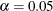CLSUM% two-sided confidence limits for the SUM, whereis determined by the ALPHA= option; the default isCV

coefficient of variation for MEAN

CVSUM

coefficient of variation for SUM

DECILES

the 10th, the 20th, …, and the 90th percentiles including their standard errors and confidence limits

DF

degrees of freedom for the t test

GEOMEAN

geometric mean of a numeric variable that contains positive values

GMCLM% two-sided confidence limits for the GEOMEAN, whereis determined by the ALPHA= option; the default isGMSTDERR

standard error of the GEOMEAN. When you request GEOMEAN, the procedure computes GMSTDERR by default.

LCLM% one-sided lower confidence limit for the MEAN, whereis determined by the ALPHA= option; the default isLCLSUM% one-sided lower confidence limit for the SUM, whereis determined by the ALPHA= option; the default isLGMCLM% one-sided lower confidence limit for the GEOMEAN, whereis determined by the ALPHA= option; the default isMAX

maximum value

MEAN

mean for a numeric variable, or the proportion in each category for a categorical variable

MEDIAN

median for a numeric variable

MIN

minimum value

NCLUSTER

number of clusters

NMISS

number of missing observations

NOBS

number of nonmissing observations

Q1

lower quartile

Q3

upper quartile

QUARTILES

lower quartile (25%th percentile), median (50%th percentile), and upper quartile (75%th percentile), including their standard errors and confidence limits

RANGE

range, MAX–MIN

STD

standard deviation of the SUM. When you request SUM, the procedure computes STD by default.

STDERR

standard error of the MEAN or RATIO. When you request MEAN or RATIO, the procedure computes STDERR by default.

SUM

weighted sum,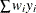, or estimated population total when the appropriate sampling weights are used

SUMWGT

sum of the weights,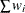T

t-value and its corresponding p-value with DF degrees of freedom for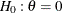, whereis a requested statistic

UCLM% one-sided upper confidence limit for the MEAN, whereis determined by the ALPHA= option; the default isUCLSUM% one-sided upper confidence limit for the SUM, whereis determined by the ALPHA= option; the default isUGMCLM% one-sided upper confidence limit for the GEOMEAN, whereis determined by the ALPHA= option; the default isVAR

variance of the MEAN or RATIO

VARSUM

variance of the SUM

See the section Statistical Computations for details about how PROC SURVEYMEANS computes these statistics.

VARMETHOD=BRR <(method-options)>
VARMETHOD=JACKKNIFE | JK <(method-options)>
VARMETHOD=TAYLOR

specifies the variance estimation method. VARMETHOD=TAYLOR requests the Taylor series method, which is the default if you do not specify the VARMETHOD= option or the REPWEIGHTS statement. VARMETHOD=BRR requests variance estimation by balanced repeated replication (BRR), and VARMETHOD=JACKKNIFE requests variance estimation by the delete-1 jackknife method.

For VARMETHOD=BRR and VARMETHOD=JACKKNIFE you can specify method-options in parentheses. Table 92.3 summarizes the available method-options.

Table 92.3: Variance Estimation Options

VARMETHOD=

Variance Estimation Method

Method-Options

BRR

JACKKNIFE

TAYLOR

Taylor series linearization

None

Method-options must be enclosed in parentheses following the method keyword. For example:

varmethod=BRR(reps=60 outweights=myReplicateWeights)


The following values are available for the VARMETHOD= option:

BRR <(method-options)>

requests balanced repeated replication (BRR) variance estimation. The BRR method requires a stratified sample design with two primary sampling units (PSUs) per stratum. See the section Balanced Repeated Replication (BRR) Method for more information.

You can specify the following method-options in parentheses following VARMETHOD=BRR:

computes the degrees of freedom as the number of nonmissing strata for an analysis variable. The degrees of freedom for VARMETHOD=BRR equal the number of strata, which by default is based on all valid observations in the data set. But if you specify the DFADJ method-option, PROC SURVEYMEANS does not count any empty strata that are due to all observations containing missing values for an analysis variable.

See the section Degrees of Freedom for more information. See the section Data and Sample Design Summary for details about valid observations.

The DFADJ method-option has no effect on categorical variables when you specify the MISSING option, which treats missing values as a valid nonmissing level.

The DFADJ method-option cannot be used when you provide replicate weights with a REPWEIGHTS statement. When you use a REPWEIGHTS statement, the degrees of freedom equal the number of REPWEIGHTS variables (or replicates), unless you specify an alternative value in the DF= option in the REPWEIGHTS statement.

FAY <=value>

requests Fay’s method, a modification of the BRR method, for variance estimation. See the section Fay’s BRR Method for more information.

You can specify the value of the Fay coefficient, which is used in converting the original sampling weights to replicate weights. The Fay coefficient must be a nonnegative number less than 1. By default, the value of the Fay coefficient equals 0.5.

H=SAS-data-set

names a SAS data set that contains the Hadamard matrix for BRR replicate construction. If you do not provide a Hadamard matrix with the HADAMARD= method-option, PROC SURVEYMEANS generates an appropriate Hadamard matrix for replicate construction. See the sections Balanced Repeated Replication (BRR) Method and Hadamard Matrix for details.

If a Hadamard matrix of a given dimension exists, it is not necessarily unique. Therefore, if you want to use a specific Hadamard matrix, you must provide the matrix as a SAS data set in the HADAMARD= method-option.

In the HADAMARD= input data set, each variable corresponds to a column of the Hadamard matrix, and each observation corresponds to a row of the matrix. You can use any variable names in the HADAMARD= data set. All values in the data set must equal either 1 or –1. You must ensure that the matrix you provide is indeed a Hadamard matrix—that is,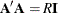, where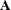is the Hadamard matrix of dimension R andis an identity matrix. PROC SURVEYMEANS does not check the validity of the Hadamard matrix that you provide.

The HADAMARD= input data set must contain at least H variables, where H denotes the number of first-stage strata in your design. If the data set contains more than H variables, the procedure uses only the first H variables. Similarly, the HADAMARD= input data set must contain at least H observations.

If you do not specify the REPS= method-option, then the number of replicates is taken to be the number of observations in the HADAMARD= input data set. If you specify the number of replicates—for example, REPS=nreps—then the first nreps observations in the HADAMARD= data set are used to construct the replicates.

You can specify the PRINTH option to display the Hadamard matrix that the procedure uses to construct replicates for BRR.

OUTWEIGHTS=SAS-data-set

names a SAS data set that contains replicate weights. See the section Balanced Repeated Replication (BRR) Method for information about replicate weights. See the section Replicate Weights Output Data Set for more details about the contents of the OUTWEIGHTS= data set.

The OUTWEIGHTS= method-option is not available when you provide replicate weights with the REPWEIGHTS statement.

PRINTH

When you provide your own Hadamard matrix with the HADAMARD= method-option, only the rows and columns of the Hadamard matrix that are used by the procedure are displayed. See the sections Balanced Repeated Replication (BRR) Method and Hadamard Matrix for details.

The PRINTH method-option is not available when you provide replicate weights with the REPWEIGHTS statement because the procedure does not use a Hadamard matrix in this case.

REPS=number

specifies the number of replicates for BRR variance estimation. The value of number must be an integer greater than 1.

If you do not provide a Hadamard matrix with the HADAMARD= method-option, the number of replicates should be greater than the number of strata and should be a multiple of 4. See the section Balanced Repeated Replication (BRR) Method for more information. If a Hadamard matrix cannot be constructed for the REPS= value that you specify, the value is increased until a Hadamard matrix of that dimension can be constructed. Therefore, it is possible for the actual number of replicates used to be larger than the REPS= value that you specify.

If you provide a Hadamard matrix with the HADAMARD= method-option, the value of REPS= must not be less than the number of rows in the Hadamard matrix. If you provide a Hadamard matrix and do not specify the REPS= method-option, the number of replicates equals the number of rows in the Hadamard matrix.

If you do not specify the REPS= or HADAMARD= method-option and do not include a REPWEIGHTS statement, the number of replicates equals the smallest multiple of 4 that is greater than the number of strata.

If you provide replicate weights with the REPWEIGHTS statement, the procedure does not use the REPS= method-option. With a REPWEIGHTS statement, the number of replicates equals the number of REPWEIGHTS variables.

JACKKNIFE | JK <(method-options)>

requests variance estimation by the delete-1 jackknife method. See the section Jackknife Method for details. If you provide replicate weights with a REPWEIGHTS statement, VARMETHOD=JACKKNIFE is the default variance estimation method.

You can specify the following method-options in parentheses following VARMETHOD=JACKKNIFE:

computes the degrees of freedom as the number of nonmissing strata for an analysis variable. The degrees of freedom for VARMETHOD=JACKKNIFE equal the number of clusters (or number of observations if there is no clusters) minus the number of strata (or one if there is no strata). By default, the number of strata is based on all valid observations in the data set. But if you specify the DFADJ method-option, PROC SURVEYMEANS does not count any empty strata that are due to all observations containing missing values for an analysis variable.

See the section Degrees of Freedom for more information. See the section Data and Sample Design Summary for details about valid observations.

The DFADJ method-option has no effect on categorical variables when you specify the MISSING option, which treats missing values as a valid nonmissing level.

The DFADJ method-option cannot be used when you provide replicate weights with a REPWEIGHTS statement. When you use a REPWEIGHTS statement, the degrees of freedom equal the number of REPWEIGHTS variables (or replicates), unless you specify an alternative value in the DF= option in the REPWEIGHTS statement.

OUTJKCOEFS=SAS-data-set

names a SAS data set that contains jackknife coefficients. See the section Jackknife Method for information about jackknife coefficients. See the section Jackknife Coefficients Output Data Set for more details about the contents of the OUTJKCOEFS= data set.

OUTWEIGHTS=SAS-data-set

names a SAS data set that contains replicate weights. See the section Jackknife Method for information about replicate weights. See the section Replicate Weights Output Data Set for more details about the contents of the OUTWEIGHTS= data set.

The OUTWEIGHTS= method-option is not available when you provide replicate weights with the REPWEIGHTS statement.

TAYLOR

requests Taylor series variance estimation. This is the default method if you do not specify the VARMETHOD= option or a REPWEIGHTS statement. See the section Taylor Series Method for more information.